# Texas Go Math Grade 3 Lesson 19.3 Answer Key Bar Graphs

Refer to our Texas Go Math Grade 3 Answer Key Pdf to score good marks in the exams. Test yourself by practicing the problems from Texas Go Math Grade 3 Lesson 19.3 Answer Key Bar Graphs.

## Texas Go Math Grade 3 Lesson 19.3 Answer Key Bar Graphs

Unlock the Problem

A bar graph uses bars to show data. A scale of equally spaced numbers helps you read the number each bar shows.

The students in the reading group made a bar graph to record the number of books they read in October. How many books did Seth read? Find the bar for Seth. It ends at books in October.

Underline the words that tell you where to find the information to answer the question.Find the bar for Seth. It ends at _________.
So, Seth read _________ books in October.
Found the bar for Seth. It ends at 8
So, Seth read 8 books in October.

Math Talk
Mathematical Processes

Explain how to read the bar that tells how many books Amy read.
Explanation:
The scale is by two from 0 to 16
The bar stopped in the middle of 8 and 10 so it is 9Question 1.
How many books did Max read?
Explanation:
The bar stopped at 6

Question 2.
Who read 4 fewer books than Kate?
Explanation:
12 – 8 = 4
Seth read 4 fewer books than Kate

Question 3.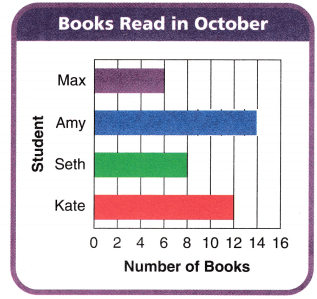Explanation:
Shaded the extra 5 on bar graph
Amy read 9 before now 5
9 + 5 = 14

Examples

These bar graphs show the same data.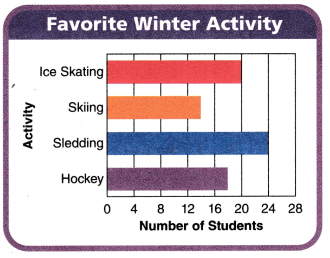In a horizontal bar graph, the bars go across from left to right. The length of the bar shows the number.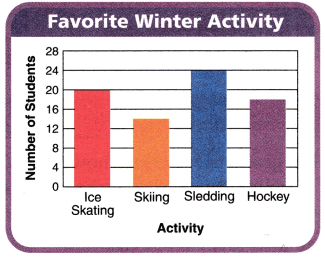In a vertical bar graph, the bars go up from the bottom. The height of the bar shows the number.

Question 4.
What does each space between the numbers represent?
Explanation:
Each space between the numbers represent a Scale

Question 5.
How can you find the winter activity that the most students voted for using the graphs above?
By seeing the bars on the scale
and the length of the bars
Most students voted for Sledding

Share and Show

Use the Spinner Results bar graph for 1-3.Question 1.
How many more times did the pointer stop on green than on purple?
________ more times
Explanation:
Number of times green is 35
Number of times purple is 20
35 – 20 = 15

Question 2.
How many fewer times did the pointer stop on blue than on red and green combined?
_________ fewer times
Number of times on red = 40
Number of times green = 35
Number of times blue = 20
Red and green are combined
40 + 35 = 75
75 – 20 = 55

Question 3.
What if there were 15 more spins and the pointer stopped 10 more times on green and 5 more times on blue? How many more times did the pointer stop on green than blue?
Explanation:
Number of times green = 35 + 10 = 45
Number of times blue = 20 + 5 = 25
45 – 25 = 20
20 more times  the pointer stop on green than blue

Problem Solving

Use the Diego’s DVDs bar graph for 4-6.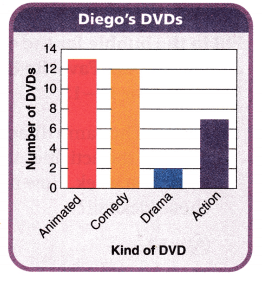Question 4.
Analyze Diego has 5 fewer of this kind of DVD than comedy. Which kind of DVD is this?
Explanation:
Diego has 5 fewer of Action kind of DVD than comedy

Question 5.
Multi-Step Is the number of comedy and action DVDs greater than or less than the number of animated and drama DVDs? Explain.
number of comedy and action DVDs = 7 + 12 = 19
number of animated and drama DVDs = 2 + 13 = 15
19-15=4

Question 6.
H.O.T. Multi-Step How many DVDs does Diego have that are not comedy DVDs?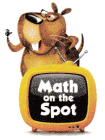Explanation:
22 DVDs does Diego have that are not comedy DVDs

Use the Science Fair Projects bar graph for 7-9.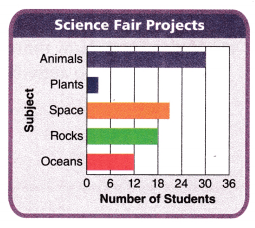Question 7.
Representations How many more students would have to do a project on plants to equal the number of projects on space?
Explanation:
Students on plants is 3
students on space is 21
21 – 3 = 18
18 more students would have to do a project on plants to equal the number of projects on space

Question 8.
Write Math What’s the Question? The answer is animals, space, rocks, oceans, and plants.
What are the subjects in the science fair project
Explanation:
What are the subjects in the science fair project, The answer is animals, space, rocks, oceans, and plants.

Question 9.
H.O.T. What if 3 fewer students did a project on weather than did a project on rocks? Describe what the bar graph would look like.Explanation:
Drawn the bar graph with the new subject weather in place of rock
Students on weather 15
students on rocks 18

Fill in the bubble for the correct answer choice. Use the Favorite Camp Activity bar graph for 10-13.Question 10.
Apply The camp director made a bar graph of the activities that campers chose last week. How many campers chose kickball?
(A) 13
(B) 9
(C) 12
(D) 8
Explanation:
9 campers chose kickball
The camp director made a bar graph of the activities that campers chose last week.

Question 11.
Multi-Step How many fewer campers chose boating and kickball combined than chose art and swimming combined?
(A) 8
(B) 3
(C) 2
(D) 17
Explanation:
campers chose boating and kickball 17
campers chose art and swimming 25
25 – 17= 8
8 fewer campers chose boating and kickball combined than chose art and swimming combined

Question 12.
Multi-Step How many campers in all chose a favorite camp activity?
(A) 30
(B) 40
(C) 29
(D) 42
Explanation:
42 campers in all chose a favorite camp activity

Texas Test Prep

Question 13.
Analyze How many campers chose a water activity?
(A) 12
(B) 18
(C) 20
(D) 8
Explanation:
Swimming and boating are the water activities
20 campers chose water activities.

### Texas Go Math Grade 3 Lesson 19.3 Homework and Practice Answer Key

Problem Solving

Use the Roxanne’s Bird Feeder bar graph for 1-4.Question 1.
Roxanne made this bar graph to show the number of birds that she saw at her bird feeder. How many more wrens than sparrows did Roxanne see?
Explanation:
Roxanne made this bar graph to show the number of birds that she saw at her bird feeder.
Roxanne see 7 more wrens than sparrows
12 – 5 = 7

Question 2.
How many fewer sparrows than robins did Roxanne see?
Explanation:
Roxanne see 3 fewer sparrows than robins
8 – 5 = 3

Question 3.
Roxanne saw 4 more of this kind of bird than robins. Which kind of bird is this?
Explanation:
Roxanne saw 4 more of  wren birds than robins

Question 4.
How many birds did Roxanne see at the feeder that were not cardinals?
Explanation:
wren, robins and sparrows birds Roxanne see at the feeder that were not cardinals.

Use the Art Fair Projects bar graph for 5-6.Question 5.
How many more students would have to make mobiles so that the number of mobiles equals the number of sculptures?
Explanation:
Number of mobiles = 8
Number of sculptures = 26
26 – 8 = 18
18 more students would have to make mobiles so that the number of mobiles equals the number of sculptures

Question 6.
List the art projects in order from the least number to the greatest number of students.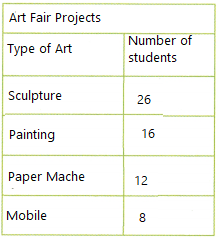Explanation:
Listed the art projects in order from the least number to the greatest number of students.
in frequency table.

Lesson Check

Fill in the bubble completely to show your answer. Use the Band Members bar graph for 7-9.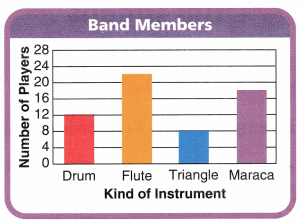Question 7.
How many more flute players than triangle players are in the band?
(A) 22
(B) 8
(C) 30
(D) 14
Explanation:
Flute players are 22
triangle players are 8
22 – 8 = 14
14 more flute players than triangle players are in the band.

Question 8.
The band needs the number of triangle players to equal the number of drum players. How many more triangle players does the band need?
(A) 4
(B) 12
(C) 8
(D) 20
triangle players are 8
number of drum players 12
The band needs the number of triangle players to equal the number of drum players.
4 more triangle players need the band

Question 9.
Multi-Step How many band members do NOT play the mazacas?
(A) 18
(B) 34
(C) 42
(D) 60
Explanation:
60 band members do NOT play the mazacas.

Use the Kenji’s Garden bar graph for 10-12.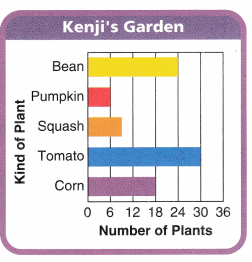Question 10.
Kenji wants to plant an equal number of corn and tomato plants. How many more corn plants does he need?
(A) 18
(B) 30
(C) 48
(D) 12
Explanation:
Number of corn plants are 18
Number of tomato plants are 30
30 – 18 = 12
Kenji wants to plant an equal number of corn and tomato plants.
Kenji wants to plant 12 more corn plants

Question 11.
Kenji plants more pumpkin plants. 12. There are double the number of pumpkin plants now. How many pumpkin plants are there?
(A) 6
(B) 9
(C) 12
(D) 15
Explanation:
Double of 6 is 12

Question 12.
Multi-Step How many fewer squash and pumpkin plants are there than corn and bean plants?
(A) 27
(B) 42
(C) 15
(D) 57